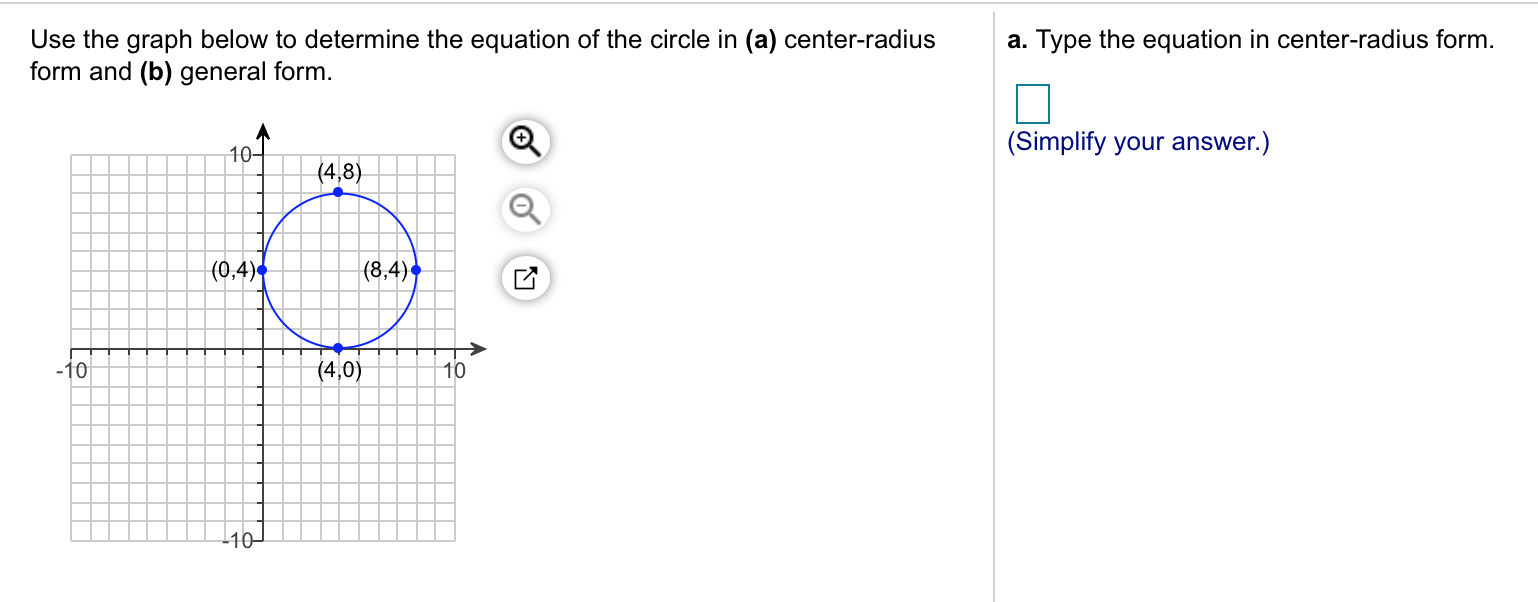# Use the graph below to determine the equation of the circle in (a) center-radiusa. Type the equation in center-radius formform and (b) general form(Simplify your answer.)10(0,4)(8,4)1010

Question
88 viewshelp_outlineImage TranscriptioncloseUse the graph below to determine the equation of the circle in (a) center-radiusa. Type the equation in center-radius form form and (b) general form (Simplify your answer.) 10 (0,4) (8,4) 10 10 fullscreen
check_circle

Step 1

Given a circle with known end points of diamemter (0,4) and (8,4), (4,0) and (4,8). The mid point of diameter is center of the circle.

Step 2

The midpoint of any two points (x1,y1) and (x2,y2) is shown below

Let us find the midpoint of given diameter ends (0,4) and (8,4) we get midpoint is (4,4). So the center of the circle is (4,4)

Step 3

The distance between end poits (4,0) and (4,8) is 8units.The diameter =8units. So the radius of circle is half the diameter which is 4 units.

The center radius form of an equation of circle with radius (h,k) and radius r is given by

(x-h)2+(y-k)2=r2

substituting (h,k)=(4,4) and r =...

### Want to see the full answer?

See Solution

#### Want to see this answer and more?

Solutions are written by subject experts who are available 24/7. Questions are typically answered within 1 hour.*

See Solution
*Response times may vary by subject and question.
Tagged in

### Algebra